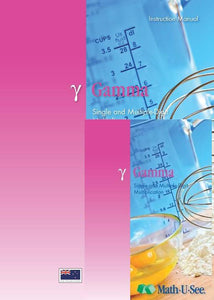or 6 weekly interest-free payments from \$12.50 withwhat's this?Demme Learning

# Math.U.See Gamma

Regular price \$65.00 \$40.63 Unit price per
Tax included. Shipping calculated at checkout.

## Math.U.See Gamma

#### Multiplication for single and multiple-digit numbers and other topics.

Once students have mastered the concepts of addition and subtraction (covered in Alpha and Beta), they are ready for multiplication. Gamma teaches single-digit facts and multiple-digit multiplication skills

Major Concepts and Skills Include:

• Using strategies based on place value and properties of operations to multiply
• Fluently multiplying any combination of whole numbers
• Solving for an unknown factor
• Solving abstract and real-world problems involving addition, subtraction, and multiplication
• Measuring and computing area
• Relating concepts of area to addition and multiplication

• Skip counting as a precursor to multiplication
• Adding and subtracting time in hours and minutes
• Multiplying, adding, and subtracting N.Z currency and standard units of measure
• Representing, recording, and interpreting data
• Understanding of basic fractions
• Estimating and solving measurement problems

Gamma Instruction Pack includes: Instruction Manual & DVD

Gamma Student pack includes: Student Workbook & Test & Activity booklet.

Grab an extra Student Pack for an additional Student.

For a complete set to get started with Math.U.See you will need to purchase the Integer Block set (a one of purchase for use with ALL levels of Math.U.See), the Gamma instruction pack & Gamma Student Pack